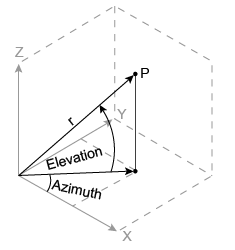# cart2sph

## 语法

``[azimuth,elevation,r] = cart2sph(x,y,z)``

## 说明

``[azimuth,elevation,r] = cart2sph(x,y,z)` 将笛卡尔坐标数组 `x`、`y` 和 `z` 的对应元素转换为球面坐标 `azimuth`、`elevation` 和 `r`。`

## 示例

`x = [1 1 1 1; -1 -1 -1 -1]`
```x = 2×4 1 1 1 1 -1 -1 -1 -1 ```
`y = [1 1 -1 -1; 1 1 -1 -1]`
```y = 2×4 1 1 -1 -1 1 1 -1 -1 ```
`z = [1 -1 1 -1; 1 -1 1 -1]`
```z = 2×4 1 -1 1 -1 1 -1 1 -1 ```
`[az,el,r] = cart2sph(x,y,z)`
```az = 2×4 0.7854 0.7854 -0.7854 -0.7854 2.3562 2.3562 -2.3562 -2.3562 ```
```el = 2×4 0.6155 -0.6155 0.6155 -0.6155 0.6155 -0.6155 0.6155 -0.6155 ```
```r = 2×4 1.7321 1.7321 1.7321 1.7321 1.7321 1.7321 1.7321 1.7321 ```

## 算法

```azimuth = atan2(y,x) elevation = atan2(z,sqrt(x.^2 + y.^2)) r = sqrt(x.^2 + y.^2 + z.^2)```